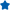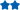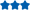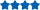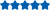• Log in
• Register
• Working Hours: Mon-Sat: EST 21:00 PM - 11:30 AM
• Oyostepper.com@gmail.com
• Cart
0
Article Category
Category
TOP Sellers
\$36.48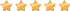\$467.84\$13.82\$10.79\$33.59\$17.99\$19.20\$27.22\$12.06\$38.10\$61.94\$9.77\$11.93\$16.80\$36.49# What is the theory of stepper motors?

A is a constant output power transducer, where power is defined as torque multiplied by speed. This means motor torque is the inverse of motor speed. To help understand why a step motor’s power is independent of speed, we need to construct (figuratively) an ideal step motor.

An ideal step motor would have zero mechanical friction, its torque would be proportional to ampere-turns and its only electrical characteristic would be inductance. Ampere-turns simply mean that torque is proportional to the number of turns of wire in the motor’s stator multiplied by the current passing through those turns of wire.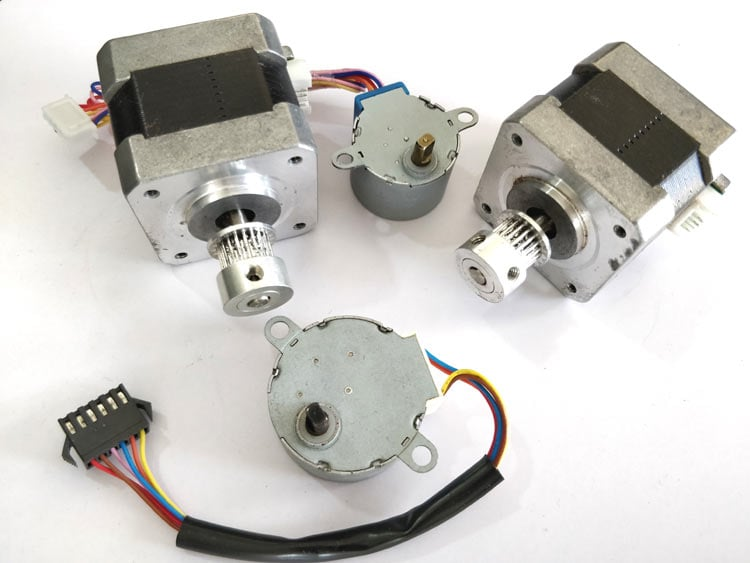Anytime there are turns of wire surrounding a magnetic material such as the iron in the motor’s stator, it will have an electrical property called inductance. Inductance describes the energy stored in a magnetic field anytime current passes through this coil of wire.

Inductance (L) has a property called inductive reactance, which for the purposes of this discussion may be thought of as a resistance proportional to frequency and therefore motor speed.

According to Ohm’s law, current is equal to voltage divided by resistance. In this case we substitute inductive reactance for resistance in Ohm’s law and conclude motor current is the inverse of motor speed.

Since torque is proportional to ampere-turns (current times the number of turns of wire in the winding), and current is the inverse of speed, torque also has to be the inverse of speed.

In an ideal step motor (nema 23 stepper motor), as speed approaches zero, its torque would approach infinity while at infinite speed torque would be zero. Because current is proportional to torque, motor current would be infinite at zero as well.

Electrically, a real motor differs from an ideal one primarily by having a non-zero winding resistance. Also, the iron in the motor is subject to magnetic saturation, as well as having eddy current and hysteresis losses. Magnetic saturation sets a limit on current to torque proportionally while eddy current and hysteresis (iron losses) along with winding resistance (copper losses) cause motor heating.

Previous:How does step motor handle inertia mismatch?
Next:NEMA 34 Stepper Motor Applications and Dimensions
No reviews
Customer reviews E-mail:

Content:
Rank: